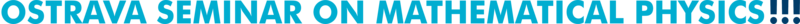Past talks (2019)
 52. February 19, 2019 Baruch Schneider (University of Ostrava) A quaternionic treatment of inhomogeneous Cauchy-Riemann type systems in some traditional theories Abstract   Slides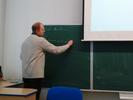53. February 27, 2019 Radosław Kycia (Krakow University of Technology and Masaryk University, Brno) Entropy, Landauer's principle and Category Theory Abstract: Usual popular-science 'definition' of entropy is: 'Entropy is some measure of disorder and disorder is what entropy measures'. In this talk I will show how to construct (and understand) the notion of entropy from first principles, that is outlined in . Then I will describe the Landauer's principle that relates irreversible operations on computer memory with heat emission from the device. It explains the Maxwell's demon paradox in thermodynamics. Finally the intriguing interplay between the Landauer's principle and the notion of the Galois connection will be provided. Some hints on application to DNA computing and biological evolution will be also given. The talk based on my recent paper . Literature:  Elliott H. Lieb, Jakob Yngvason, A Guide to Entropy and the Second Law of Thermodynamics, Notices Amer. Math. Soc. 45 (1998), 571-581  Radosław A. Kycia, Landauer's Principle as a Special Case of Galois Connection, Entropy 20 (2018), no.12, 971 Slides54. February 28, 2019 David Saunders (University of Ostrava) Lagrangians with reduced-order Euler-Lagrange equations Abstract: Any Lagrangian form of order k obtained by horizontalization of a form of order k-1 gives rise to Euler-Lagrange equations of order strictly less than 2k. But these are not the only possibilities. For example, with two independent variables, the horizontalization of a first-order 2-form gives a Lagrangian quadratic in the second-order variables; but there are also cubic second-order Lagrangians with third-order Euler-Lagrange equations. In this talk I shall show first that any Lagrangian of order k with Euler-Lagrange equations of order less than 2k must be a polynomial in the k-th order variables of order not greater than the number of different symmetric multi-indices of length k. I shall then describe a geometrical construction, based on Peter Olver's idea of differential hyperforms, which gives rise to Lagrangians with reduced-order Euler-Lagrange equations. A version of this talk was given at Ostrava in June 2017. The work has been published in SIGMA 14 (2018), 089, 13 pages. Slides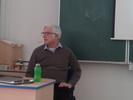55. March 6, 2019 Pasha Zusmanovich (University of Ostrava) Lie algebras and around: selected questions We will provide an idiosyncratic survey of some interesting open questions in Lie algebras and related structures. The topics include Lie algebras with given properties of subalgebras, Lie algebras of cohomological dimension one, peculiarities in characteristic 2, and some odd cousins dubbed omega-Lie and Hom-Lie algebras. Most of the topics will be accessible to unenlightened students. 56. March 20, 2019 Igor Khavkine (Institute of Mathematics of the Czech Academy of Sciences, Prague) IDEAL characterization of cosmological and black hole spacetimes Abstract: On a (pseudo-)Riemannian manifold $$(M,g)$$, an IDEAL characterization of a reference geometry $$(M_0,g_0)$$ consists of a list of tensors $$\{T_i[g]\}$$ locally and covariantly constructed from the metric $$g$$, such that $$T_i[g] = 0$$ iff $$(M,g)$$ is locally isometric to $$(M_0,g_0)$$. Unfortunately, to date only a few IDEAL characterizations are known for interesting geometries. But if known, they have interesting applications to analysis and geometry on the reference background $$(M_0,g_0)$$. I will discuss how such characterizations were recently obtained for a class of cosmological and black hole spacetimes. Slides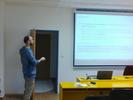57. March 21, 2019    Aleksander Iwanow (Silesian University of Technology, Gliwice) Pseudocompact structures of unitary representations of finitely generated groups Abstract: We apply methods of continuous logic to unitary representations of finitely generated groups. In this approach Hilbert spaces are considered as many-sorted continuous metric structures. Unitary representations arise as expansions of these structures by unitary operators. A structure is pseudocompact if it is elementarily equivalent to a metric ultraproduct of compact ones. We connect pseudocompactness with the topic of approximations by metric groups. In particular we consider property MF for finitely generated groups. Literature: Continuous theory of operator expansions of finite dimensional Hilbert spaces, continuous structures of quantum circuits and decidability, arXiv:1805.03070.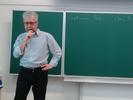58. March 27, 2019 Orif Ibrogimov (Czech Technical University in Prague) Spectrum of the truncated spin-boson Hamiltonian for large coupling Abstract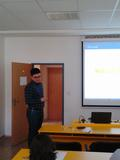59. April 24, 2019 Přemysl Jedlička (Czech University of Life Sciences, Prague) Set theoretic solutions of Yang-Baxter equation and retraction congruence Abstract: Yang-Baxter equation is an equation from particle physics. A set-theoretic solution of Yang-Baxter equation is a bijection $$r:X^2\to X^2$$ satisfying $(r \times id) (id \times r) (r\times id) = (id \times r) (r \times id) (r\times id).$ A solution is called non-degenerate, if, for $$r=(\sigma_x(y),\tau_y(x))$$, the mappings $$\sigma_x$$ and $$\tau_y$$ are permutations. A solution is called involutive if $$r^2=id$$. There is a much-studied congruence of a non-degenerate involutive solution, called the retraction, denoted by $$\sim$$ and defined as $$x\sim y$$ if and only if $$\sigma_x=\sigma_y$$. We present a generalization of this congruence, defined for non-involutive solutions.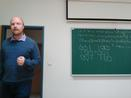60. May 15, 2019 Jiří Lipovský (University of Hradec Králové and Nuclear Physics Institute, Czech Academy of Sciences) Non-Weyl quantum and microwave graphs Abstract: An interesting question is to count the number of resolvent resonances of quantum graphs which are encircled in the circle of radius $$R$$ in the complex plane in the limit for $$R\to \infty$$. Davies and Pushnitski found that for some graphs this number grows with $$R$$ slower than one would expect from the Weyl asymptotics. We will introduce criteria on how to distinguish the non-Weyl graphs from the Weyl ones and explain why this phenomenon occurs. Recently, this behavior was experimentally verified using microwave graphs; we will give details of this experiment. The talk will be based on joint works with E.B. Davies, P. Exner, M. Lawniczak and L. Sirko. Slides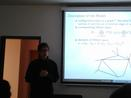61. May 16, 2019 Wolfgang Alexander Moens (University of Vienna) Groups admitting an automorphism that satisfies a polynomial identity Abstract: Suppose that $$G$$ is a group and that $$f$$ is one of its automorphisms. What can we say about the structure of $$G$$ if we know that, for all $$x \in G$$, we have a polynomial identity of the form $$x \cdot f(x^{-1}) \cdot f^2(x) = 1$$ or $$x^3 \cdot f(x) = 1$$ or $$x^{12} = 1$$? Questions such as these naturally appear in the context of the Gorenstein-Herstein conjecture, the Frobenius conjecture, the Burnside problems, and the study of universal power automorphisms. We will illustrate how some of these classical results can be extended to describe the rough structure of the groups mentioned in the title. Under reasonable assumptions, such a group $$G$$ will be solvable. And, under slightly stronger assumptions, the group $$G$$ will even be nilpotent of a certain class. Slides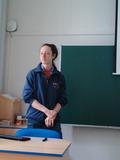62. September 11, 2019 Ivan Kaygorodov (Federal University of ABC, Santo André, Brazil) About some generalizations of a theorem of Moens Abstract: Jacobson (1955) proved that every finite-dimensional Lie algebra (over a field of characteristic 0) with an invertible derivation is nilpotent. Later, Moens (2013) generalized this result for Leibniz-derivations. We will talk about some possible generalizations of Jacobson's and Moens' theorems for another non-associative algebras (Malcev, Jordan, alternative and other). Slides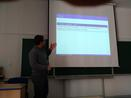63. September 25, 2019 Alexei Stepanov (I. Kant Baltic Federal University, Kaliningrad, Russia) Mathematical theory of records Abstract: In the present talk, we discuss the concept of record times and values and the ties between record values and maxima. We reveal problems and applications, which lead us to the necessity to study records. Then we present distributional results for record values and times in the continuous case. Making use of the distributional results, we state limit results for records in the continuous case. We then discuss distributional and limit results for discrete records. We also introduce the concept of weak records. We tell about similarities and differences between records and weak records. We present algorithms of generation of continuous records. Then we consider some statistical procedures related to records. In the end of the talk, we take up the notion of bivariate records. Slides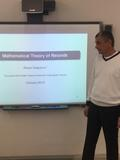64. October 22, 2019 Viktor Lopatkin (St Petersburg University) Braid spirit of Young tableaux Abstract   Slides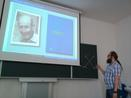65. October 23, 2019 Sergei Ivanov (St Petersburg University) Bousfield's localization of groups Abstract: In the beginning of 70's Sullivan developed a theory of localizations of simply connected spaces. Later Bousfield developed a generalization of this theory for all spaces. It turned out that this this theory is much more complicated and it is difficult to control homotopy groups of the localization when the original space in not simply connected. It is difficult to control even the fundamental group of the localization. However Bousfield proved that the fundamental group of localization depends only on the fundamental group of the original space. The corresponding functor on the category of groups is called HR-localization. We give an introduction the the theory of HR-localizations of groups, discuss open problems and our results in this theory. Slides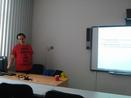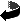Ostrava Mathematical Seminar
Created: Tue Feb 19 2019